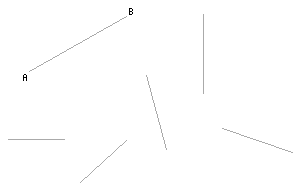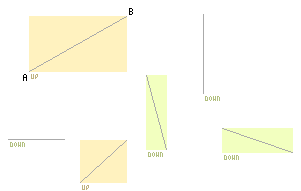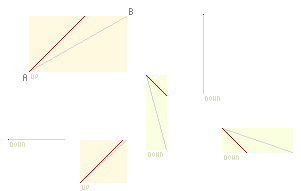# DRAWING LINES IN JAVASCRIPT

698 words ~ 3-6 mins — Mathieu 'p01' Henri on November 3rd, 2001

# Drawing lines in JavaScript

An efficient method to draw and animate many lines in JavaScript, without Canvas or any similar graphic API.

## The problem

You probably already said to yourself : "It'd be great if I could draw and move some lines at will in JavaScript." If you haven't, let's pretend you did.We want to draw a line from A to B. The position of both points may vary.

## The common technique

The first idea that may strike you is to generate a serie of DIV of 1px per 1px to draw the line.

``````var bla = ""
var lineLength = Math.sqrt( (Ax-Bx)*(Ax-Bx)+(Ay-By)*(Ay-By) );
for( var i=0; i<lineLength; i++ )
{
bla += "<div style='position:absolute;left:"+ Math.round( Ax+(Bx-Ax)*i/lineLength  ) +"px;top:"+ Math.round( Ay+(By-Ay)*i/lineLength  ) +"px;width:1px;height:1px;background:#000'></div>";
}
document.body.innerHTML += bla;``````

Congratulations! It works, it's dead simple and extremely short. Alas it's slow and generates a hell lot of useless tags, not to mention the difficulty to move a line.

Of course it can be optimized a bit using some Bresenham line algorithms and making use of symetry, etc... but it solves partially the cons of this method and is still not fast enough for realtime animation.

Now let's use our eyes and brain.

## An efficient method to draw lines in JavaScript

The key to get a fast script, is to do the minimum. Trying to figure the common factors of the lines we saw above will lead us in that direction.As you can see the lines:

• are enclosed in a box
• go either up or down ( from left to right )
• have a small side and large side

Try to project mentally the lines on the small edge of their bounding box. It should ring a bell.

Shazam! Any line can be reproduced by stretching a diagonal. If you need more to be convinced, just look the figure below.So what we need is to figure the width and height of the bouding box, and the direction of the line and size of the small edge.

Since we'll stretch some images, we must take care to 2 things:

• that the browser won't have too resize them to an unnecessary big size
• that we won't waste too much disk space or bandwidth with the images of the diagonnals

Both things can be considered if we only use some diagonnals whose size is a power of 2, that is : 1, 2, 4, 8, 16, 32, 64, ... Ok, it will introduce some small stretching artifacts but that's a small price to pay to move the lines in realtime.

Getting the position of the bounding box and the size of its smaller edge is staight forward. Calculating the direction of the line corresponds more or less to compute in which quadrant of a circle it is pointing.

### Implementation of the "image stretching" line drawer

``````function drawLine( lineObjectHandle, Ax, Ay, Bx, By, lineImgPath )
{
/*
*    lineObjectHandle = an IMG tag with position:absolute
*/
var
xMin        = Math.min( Ax, Bx ),
yMin        = Math.min( Ay, By ),
xMax        = Math.max( Ax, Bx ),
yMax        = Math.max( Ay, By ),
boxWidth    = Math.max( xMax-xMin, 1 ),
boxHeight   = Math.max( yMax-yMin, 1 ),
tmp         = Math.min( boxWidth, boxHeight ),
smallEdge   = 1,
newSrc;

while( tmp<=1 )
smallEdge<<=1;

newSrc = lineImgPath+ smallEdge +( (Bx-Ax)*(By-Ay)<0?"up.gif":"down.gif" );
if( lineObjectHandle.src.indexOf( newSrc )==-1 )
lineObjectHandle.src = newSrc;

with( lineObjectHandle.style )
{
width   = boxWidth  +"px";
height  = boxHeight +"px";
left    = xMin      +"px";
top     = yMin      +"px";
}
}``````

In the end, that method is not that tricky and needs a single IMG tag per line.

### Notice

Pixel perfect precision can be achieved at the price of having all possible diagonals. It takes a lot more time, and connections, to preload the images but the end result look nicer and is slightly faster than the quirk method above.

Clipping is not an option as it will require to touch more CSS properties.

### Applications

The number of applications is endless: route annotations on maps, drawing app, measuring tool, games, ...